# Graphic Calculations

The following article is from The Great Soviet Encyclopedia (1979). It might be outdated or ideologically biased.

## Graphic Calculations

methods of deriving numerical solutions of various problems by graphic constructions. Graphic calculations (graphic multiplication, graphic solution of equations, graphic integration, and so forth) consist in a system of constructions that duplicate or approximate analytic operations. Graphic realization of these operations relies on a sequence of constructions that eventually leads to the graphic determination of the unknown quantity. Graphs of functions are used in graphic calculations. Graphic calculations are used extensively in applied mathematics. Their advantages are methodological simplicity and ease of visualization. The major drawback is the lack of accuracy in the obtained solutions. However, in many problems, especially in engineering, the degree of accuracy of graphic calculations is quite sufficient. Graphic calculations can be successfully used to obtain first approximations that are then made more precise analytically. Sometimes calculations relying on nomograms are considered graphic calculations. This is not quite accurate since nomograms are geometric representations of functional relations and. when used to find numerical values of a function, require no constructions.

Calculations of algebraic expressions. In graphic calculations numbers are usually represented as directed segments on a line. A unit segment is selected, which serves as the scale of the graph. One direction on the line is taken to be positive. Segments representing the positive numbers are laid off in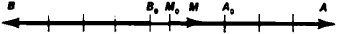Figure 1. Representation of the numbers 1, 3, and -4 by directed segments on a line

this direction; negative numbers are represented by segments laid off in the opposite direction. In Figure 1. the segments M0M, A0A, and B0B correspond to the numbers 1,3, and -4. (Here, the positive direction is to the right.)

To find the sum of two numbers, we place the corresponding segments one after the other on the line, so that the initial point of the second segment coincides with the terminal point of the first. The sum is represented by the segment whose initial point is the initial point of the first segment and whose terminal point is the terminal point of the second. To find the difference between two numbers we form the sum of the segment representing the first number, and the segment opposite the segment representing the second number.

Multiplication and division are performed by using proportional segments, which are cut off by parallel lines on the sides of an angle (MA and BC in Figure 2). Segments I. a, b.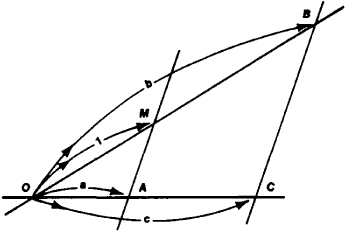Figure 2. Graphic multiplication and division: c — ab, b — c/a

and c are constructed in this manner, and their lengths satisfy the ratio a: 1 = c: b, from which c = ab or b = c/a. Hence, knowing the length of two of the three segments a, b, and c, it is always possible to find the length of the third, that is, it is possible to construct the quotient or product of two numbers. The unit segments on the lines OB and OC need not be the same.

Combining the operations of addition and multiplication, we can graphically calculate such expressions as

a1x1 + a 2x 2 + ... + an xn

and the weighted mean

(a1x1 + ... +an x n)/(a1 + ... + an

Raising a quantity to a whole higher power can be graphically done by repeated multiplication. Graphic calculation of the values of a polynomial

f(x) = a0xn + a1xn-1 + ... + an-1x + an

is based on expressing the polynomial in the form

f(x) = {[(a0xn+ a1)x + a2] x +... } x + an

and successively graphically executing operations, beginning with the expression given in the innermost parentheses.

An equation f(x) = 0 is graphically solved by graphing the function y = f(x) and finding the abscissas of the points of intersection of the curve with the x-axis. This gives the values of the roots of the equation. Sometimes the solution can be greatly simplified by expressing the equation in the form Φ1 (x) = Φ2(x) and graphing the curves y1(x) and y = Φ2(x). The roots of the equation are the values of the abscissas of the points of intersection of the two curves. (Figure 3 shows how the root is found.)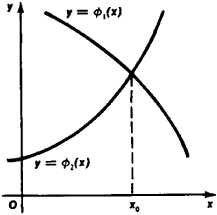Figure 3. Graphic solution of equation Φ 1(x) — Φ2(x)

Thus, to solve a cubic equation z3 + az2 + bz + c = 0, we rewrite the equation in the form x3 + px + q = 0 by replacing z with x - a/3. Then the latter equation is written in the form x3 = -px - q, and the curve y = x3 and the line y = -px - q are graphed. The points of intersection give the roots x1, x2, and x3 This construction is very convenient inasmuch as the cubic parabola y = x3 remains constant for all cubic equations. The equation.x3 - 2.67x- 1 = 0 is solved in Figure 4.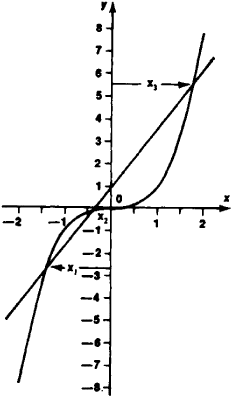Figure 4. Graphic solution of the cubic equation x>— 2.67x —1 = 0

Its roots are x1= -1.40, x2 = -0.40, and x3 = 1.80. Solutions of fourth-degree equations z4 + az3 + bz2 + cz + d = 0 can be found in the same manner. The substitution x -a/4 reduces the equation to an equation of the form x4 + px2 + qx + s =0, The latter equation is equivalent to the system of equations y = x2 and (x - x0)2+(y-y)02= r2 where x0 = -q/2, y 0 = (1 -p)/2. and r =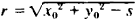The first equation is a parabola (which is the same for all fourth-degree equations), and the second, a circle with radius r and center at(x0, y0); the coordinates of the center are easy to calculate from the coefficients of the given equation.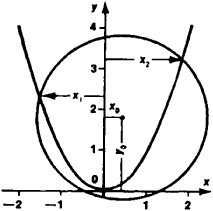Figure 5. Graphic solution of the quartic equation x4—2.6x2-0.8x -0.6 = 0

Figure 5 shows the solution of the equation x4 - 2.6x2 -O.8x - 0.6 = 0 (for this equation, x0 = 0.4, y0 = 1.8, and r = 2). The roots of the equation area:, = -1.55 andx2 =1.80. As is apparent from the figure, the equation has no other real roots.

Graphic integration. The evaluation of a definite integral fjix) dx is based on replacing the graph of the integrand y = f(x) with a step curve. Figure 6, a shows a curvilinear trapezoid aABb, whose area is numerically equal to the integral being evaluated. To construct the required step curve, the curvilinear trapezoid is divided by a series of lines, parallel to the y-axis, into a series of strips—elementary curvilinear trapezoids. Now in each strip the segment of the curve is replaced by a line segment parallel to thex-axis such that the resultant rectangles take up approximately the same area as the elementary curvilinear trapezoids. (The step curve is shown in Figure 6, a by the bold curve.) The area bounded by the step curve is equal to the sum of the area of the rectangles, that is.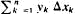where Δxk is the length of the base of the kth rectangle and its height y* is one of the values of the function >• =f{x) on the segment Δλ>. This expression gives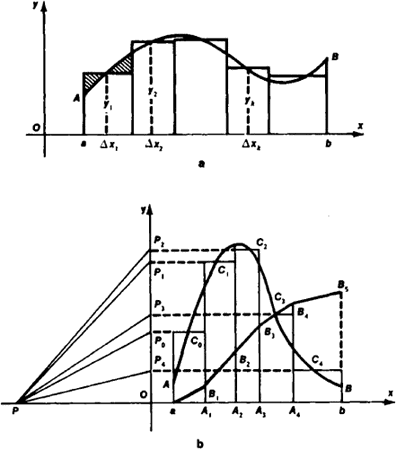Figure 6. Graphic integration an approximate value for the integral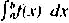The sum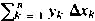ykΔxk can be calculated graphically in the manner discussed above. Figure 6.b shows all the constructions needed to calculate the integral(where the function y=f(x) is given by the graph AC0C4B. After dividing the curvilinear trapezoid by lines passing through the points A, .....3,A4 one constructs the rectangles. Their heights, the ordinates of the points C0.....C4, are laid off on the y-axis. The resulting points P0.....P4 are joined to the p point P (OP = 1). Then, beginning with the point a, the polygonal curve aB1, . . . Bs is drawn, the segments of which are parallel to the corresponding segments PP0PP,1.....PP4 The integral is numerically equal to the ordinate of point B5. To construct the graph of the antiderivative of the function y= f(x) (that is, one connects the points of the polygonal curve obtained when calculating(the points B0,B,1......B5 in Figure 6,b), by a smooth curve.

Graphic differentiation. The graph of a derivative can be constructed using the slopes of the tangents to the graph of the given function at its various points. This method is not very precise due to large errors in determining the directions of the tangent lines. The graph of a derivative can also be constructed using secants and repeating in reverse order the process of graphic integration depicted in Figure 6,b. For this purpose we draw lines parallel to the y-axis and separated by a fixed distance. Ax These lines intersect the graph of the functions in points A1, A2 (Figure 7). Then we draw segments AB1, A1B 2, . . parallel to the x-axis. The segments B1A A1, B2A A2, are equal to the corresponding increments of the function. These segments are now drawn from thex-axis. A step curve is constructed through the resulting points A1A2..... Now a curve is drawn such that the curvilinear triangles within a given step have equal areas. This curve is the graph of the derivative.

Graphic integration of differential equations. A differential equation of the first order dy/dx = f(x, y) defines a direction field in the plane. The problem of integrating the equation consists in drawing curves whose tangents have the same direction as the field. Various methods of graphic integration amount to stepwise construction of integral curves, whose tangents have prescribed slopes. To a large extent these methods duplicate the numerical methods of integration.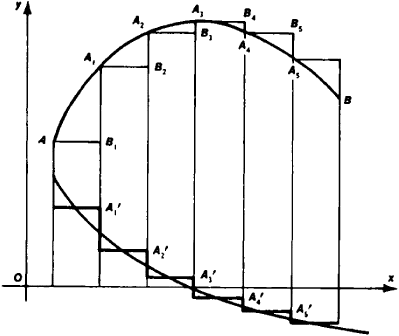Figure 7. Graphic differentiation

### REFERENCES

Golovnin, D. N. Graficheskaia matemalika. Moscow-Leningrad, 1931.
Runge. K. Graficheskie melody malematicheskikh vychislenii. Moscow-Leningrad, 1932. (Translated from German.)

M. V. PENTKOVSKII